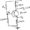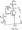# Electronic Circuits January 2007

Note : 1 Answer any FIVE full questions.

2. Missing data may be suitably assumed.

1 a. Explain diffusion capacitance. Obtain an expression for the diffusion capacitance in a P-N diode.

b.  What is a voltage multiplier circuit? Explain the operation of a full wave voltage doubler circuit.

c.  Assuming ideal diode in the circuit shown below, draw the output voltage for the given input signal.2 a. Draw and explain, a double diode clipper circuit, which limits the output at two independent levels.

b.  Explain how a diode can be used in a transistor to compensate for changes in IC0

c.  For the circuit shown in the Fig.2(c) determine Ic, Vce, Ri, Vb.Fig.2(c)

3 a. Explain how h-parameters can be obtained from the static characteristics of a transistor.

b.  State and explain Millers theorem.

c.  For the common emitter amplifier with collector to base bias shown in the Fig.3(c) Calculate : Aj, R’, Av and Avs

Given : hie = 1.1 k ; hfl = 50 h« = 25 ,uA/V ; hre = 2.5X10-44 a. Draw the hybrid – n model of a transistor and explain the significance of each component in the model.

b.  Derive expressions for transistor transconductance gm and input conductance gb,e.

c.  Explain the various types of distortions encountered in amplifiers.

5 a. What is negative feedback in amplifiers? Show that negative feedback increases the bandwidth of an amplifier.

b. Derive an expression for the input resistance with feedback amplifier employing voltage series feedback.

c. An amplifier with negative feedback has voltage gain 120. It is found that without feedback an input signal of 60mV is required to produce a particular output, whereas with feedback the input signal must be 0.5V to get the same input. Find Av and p of the amplifier.

6 a. Show that a transformer coupled class-A amplifier has a maximum power efficiency of 50%.

b. With circuit diagram explain the working of class-B push-pull amplifier. Also obtain an expression for the maximum conversion efficiency of this amplifier.

C. How much maximum power can be dissipated in the individual transistors of sl‘ class-B push-pull power amplifier if Vcc = 20V and RL – 4Ω.

7 a. With usual notations derive an expression for the voltage gain of a practical inverting Op-amp.

b. Describe a method of measuring and calculating CMRR of an op-amp.

c. What are the advantages of active filters over passive ones? Design a first order high pass filter at a cutoff frequency of 10 kHz with a pass band gain of 1.5.

8 a. Draw an inverting op-amp Schmitt trigger circuit and explain its working.

b.  Explain the principle of operation of a R-2R ladder type D to A converter.

c.   With the help of a neat diagram and relevant waveforms explain the working of a monostable multivibrator circuit using 555 timer.